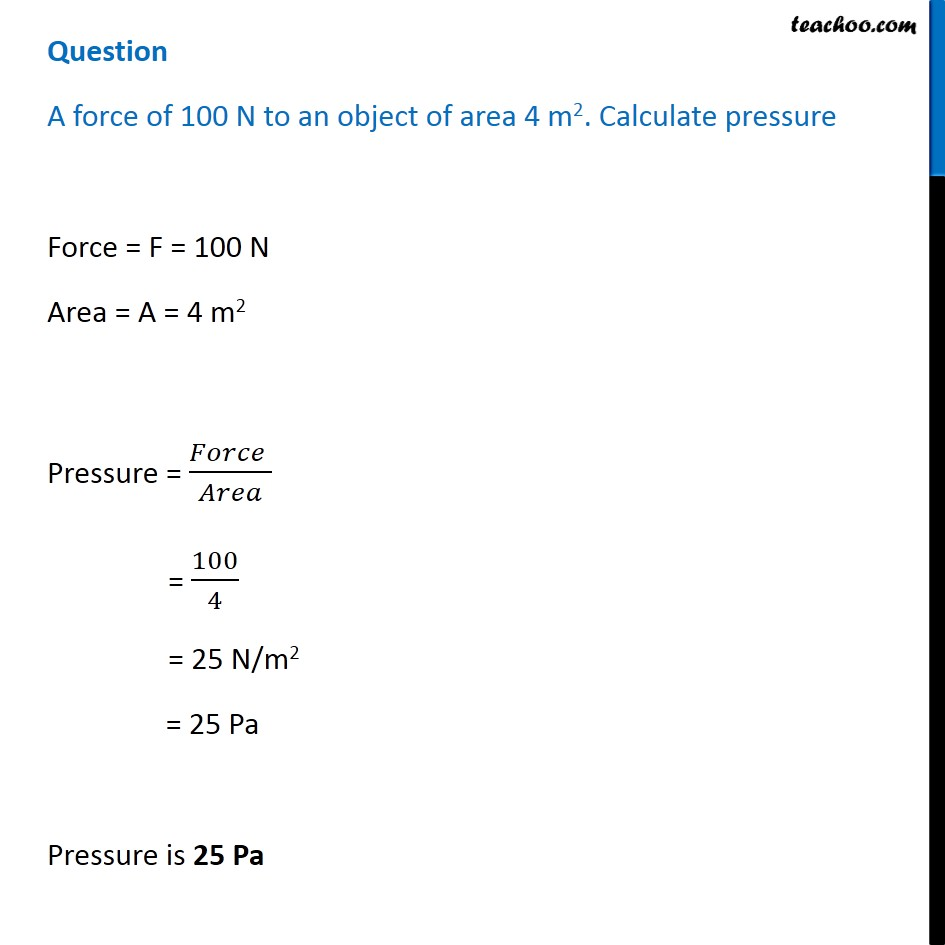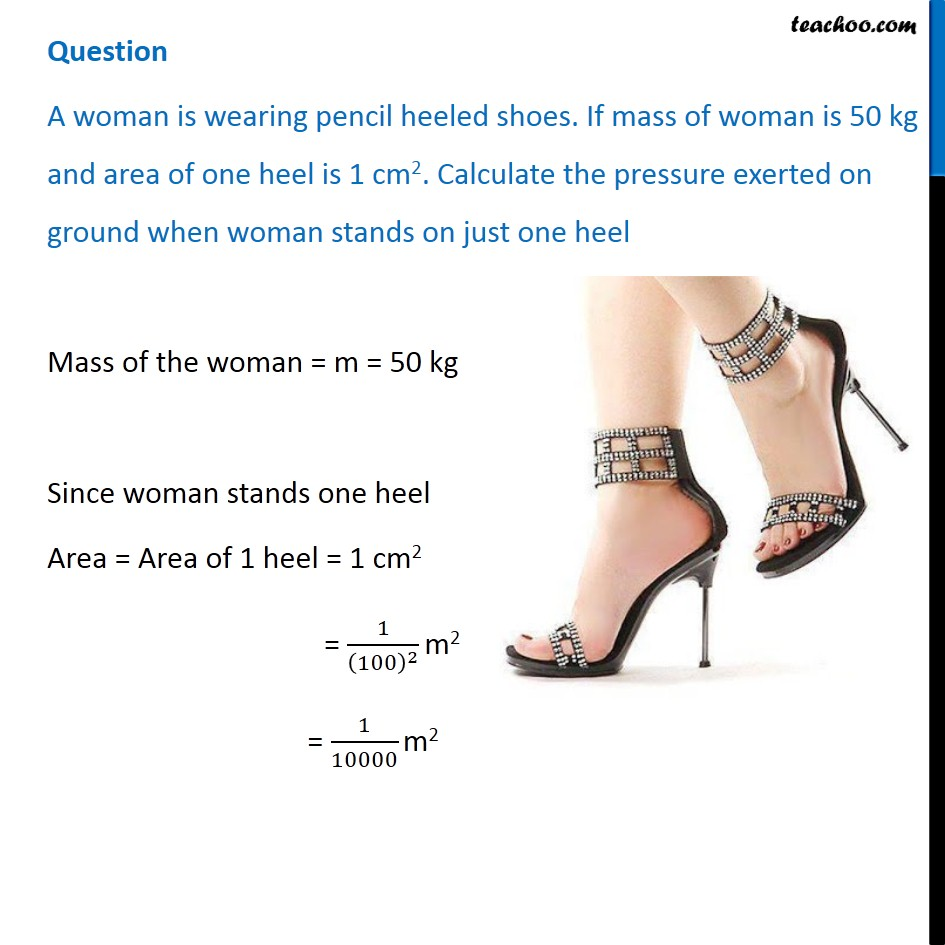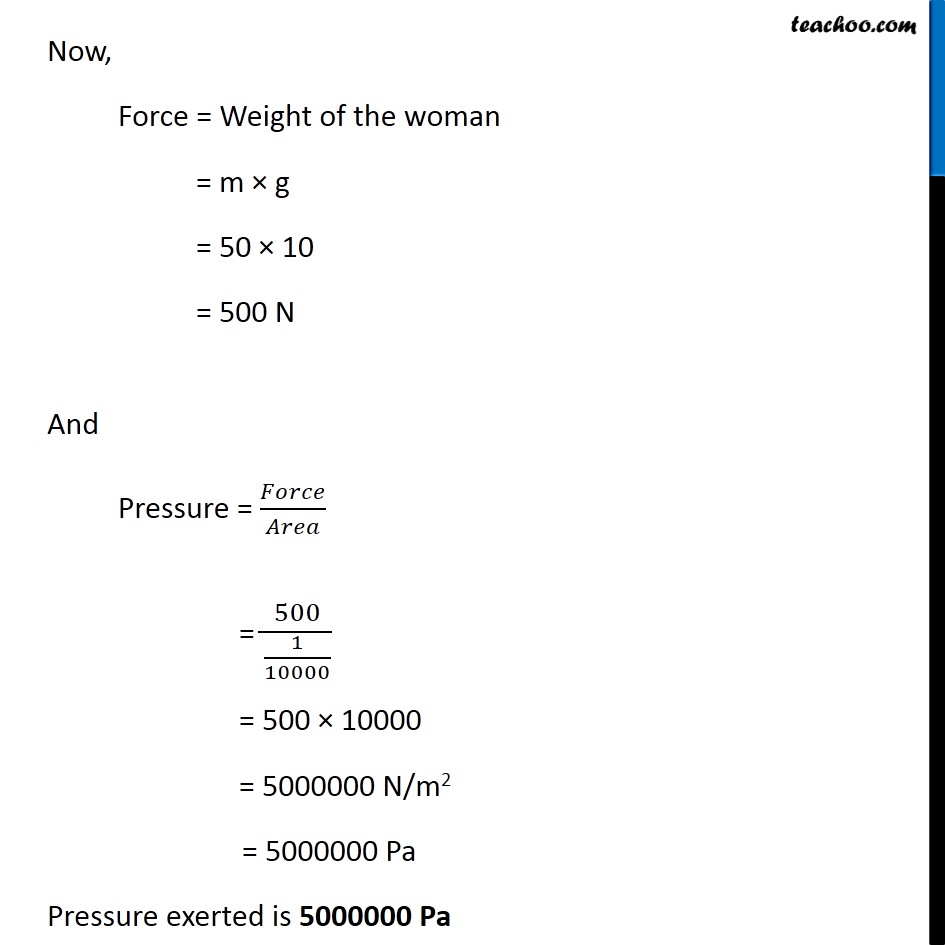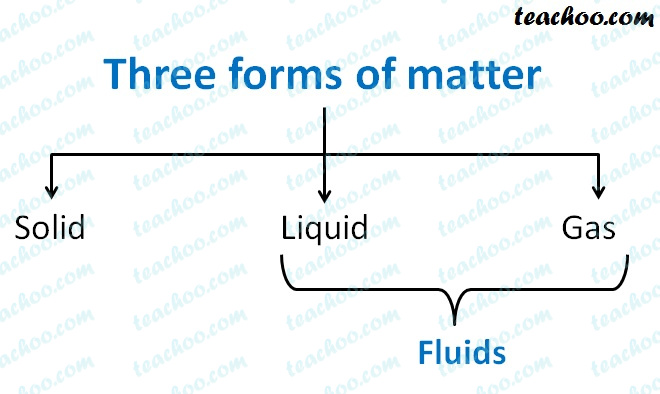Concepts

Class 9
Chapter 10 Class 9 - Gravitation

Pressure is force acting per unit of area

Pressure = Force (Thrust) / Area

Note

Lesser the area,more the pressure

More the area,less the pressure

## Suppose a square box of mass 100 kg is placed on ground

It has a width of 5 meters

What is Thrust(Force) and Pressure

Take value of g to be 10 m/s 2

Ans

Mass = m = 100 kg

g = 10 m/s 2

Thrust = m × g

= 100 × 10

= 1000 Newtons

Side of square box = 5 meters

Area of square box = 5 × 5 = 25 m 2

Pressure = Force/Area

= 1000/25

= 40 N/M 2

= 40 Pa

## What happens if we reduce size of box to 2 meters

Suppose a square box of mass 100 kg is placed on ground

It has a width of 2 meters

What is Thrust(Force) and Pressure

Take value of g to be 10 m/s 2

Mass = m = 100 kg

g = 10 m/s 2

Thrust = m × g

=100 × 10

= 1000 Newtons

Side of square box = 2 meters

Area of square box = 2 × 2 = 4 m 2

Pressure = Force/Area

= 1000/4

= 250 N/M 2

= 250 Pa

(Note SI unit of Pressure is Pascal ,denoted by Pa)

Summary

Lesser the area,more the pressure

More the area, less the pressureExamples

When we stand on loose sand,our feet sink into it

But when we lie down, we do not sink

This is because when we are standing,

Force of our body is exerted through our feet

which have smaller area

and hence more pressure is exerted on sand

But when we are lying

More area of our body is in contact with stand

Since Area increases, Pressure is reduced

So our body does not sink

## Pressure in Fluids

### What are 3 forms of Matter

Three forms of Matter are

• Solid (Example - Ice)
• Liquid (Example - Water)
• Gas (Example - Water Vapor)

#### What are fluids ?

it is a common name given to liquids and gases.

So liquid and gases are both called fluids## Is pressure exerted by fluids

yes

All forms of matter exert pressure

Just like solids have weight and exert pressure

Similarly fluids also have weight and exert pressure

## Examples of Fluid Exerting Pressure

Suppose we fill a balloon with water, it bulges. This is because of pressure of water on surface of balloon

Similarly if we fill a balloon with air, it bulges. This is because of pressure of air on surface of balloon

Pressure = Force/ Area

Hence pressure is inversely proportional to the area in contact.

Let's look at some example of pressure

## School bags have wide straps

Pressure = Force/ Area

Hence pressure is inversely proportional to the area in contact.

If the straps of the bag would not be wide, the pressure exerted on the shoulders would be large.

Therefore, straps of school bags are made wide in order to reduce the pressure exerted on the shoulders.

## Sharp knife cuts better than a blunt knife.

A sharp knife has a very thin edge as compared to a blunt knife.

When we cut an object using a sharp knife, the force is exerted on a very less area.

Since the area of contact is less, the pressure exerted is very large.

While, in case of a blunt knife the area in contact is relatively more and hence the pressure exerted is less.

Hence, a sharp knife cuts better than a blunt knife.

## Tip of a needle is sharp

The tip of a nail is sharp because the sharper the tip, the lesser will be the area of the tip.

When the needle would be hammered, the large force exerted by the hammer would fall on the very small area of the tip.

Due to small area of the needle in contact with the object, the pressure exerted on the object would be very large.

Hence, the needle would pierce in to the object easily.

## Pressure on ground is more when we are walking than when we are standing

When we are standing, both are feet are in contact with the ground while when we are walking, one of our feet is always in air.

Hence, the area in contact with the ground is more when we are standing than when we are walking.

Greater the area in contact, lesser would be the pressure.

Hence, pressure on ground is more when we are walking than when we are standing.

## Depression is much more when a man stands on a cushion than when he lies down.

When a man is standing on a cushion the area in contact is much less than when he lies down.

Lesser the area in contact, more would be the pressure.

Hence, the pressure would be more when the man is standing on the cushion than when he is lying on it.

Tractors have broad tyres, hence the area in contact with the ground is large.

Due to this large area in contact, the pressure exerted would be less.

Hence, the tyres of the tractors don not sink into comparatively soft ground in fields.

## Questions

Q 1 Page 141 - Why is it difficult to hold a school bag having a strap made of a thin and strong string?

Example 10.6 - A block of wood is kept on a tabletop. The mass of wooden block is 5 kg and its dimensions are 40 cm × 20 cm × 10 cm. Find the pressure exerted by the wooden block on the table top if it is made to lie on the table top with its sides of dimensions (a) 20 cm × 10 cm and (b) 40 cm × 20 cm.

Learn in your speed, with individual attention - Teachoo Maths 1-on-1 Class

### Transcript

Question A force of 100 N to an object of area 4 m2. Calculate pressure Force = F = 100 N Area = A = 4 m2 Pressure = (𝐹𝑜𝑟𝑐𝑒 )/𝐴𝑟𝑒𝑎 = 100/4 = 25 N/m2 = 25 Pa Pressure is 25 Pa Question A force of 100 N to an object of area 4 m2. Calculate pressure Force = F = 100 N Area = A = 4 m2 Pressure = (𝐹𝑜𝑟𝑐𝑒 )/𝐴𝑟𝑒𝑎 = 100/4 = 25 N/m2 = 25 Pa Pressure is 25 Pa Now, Force = Weight of the woman = m × g = 50 × 10 = 500 N And Pressure = 𝐹𝑜𝑟𝑐𝑒/𝐴𝑟𝑒𝑎 = 500/(1/10000) = 500 × 10000 = 5000000 N/m2 = 5000000 Pa Pressure exerted is 5000000 Pa